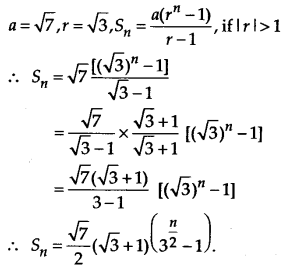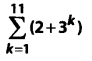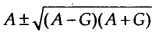NCERT Solutions for Class 11 Maths Chapter 9 Sequences and Series Ex 9.3

# NCERT Solutions for Class 11 Maths Chapter 9 Sequences and Series Ex 9.3

## NCERT Solutions for Class 11 Maths Chapter 9 Sequences and Series Ex 9.3

NCERT Solutions for Class 11 Maths Chapter 9 Sequences and Series Ex 9.3 are the part of NCERT Solutions for Class 11 Maths. Here you can find the NCERT Solutions for Class 11 Maths Chapter 9 Sequences and Series Ex 9.3.

### Ex 9.3 Class 11 Maths Question 1.

Find the 20th and nth terms of the G.P. 5/2, 5/4, 5/8, ….

Solution:

### Ex 9.3 Class 11 Maths Question 2.

Find the 12th term of a G.P. whose 8th term is 192 and the common ratio is 2.

Solution:
We have, a8 = 192 and r = 2.

### Ex 9.3 Class 11 Maths Question 3.

The 5th, 8th and 11th terms of a G.P. are p, q and s, respectively. Show that q2 = ps.

Solution:
We are given that

### Ex 9.3 Class 11 Maths Question 4.

The 4th term of a G.P. is square of its second term, and the first term is –3. Determine its 7th term.

Solution:
Given, a =  -3, a4 = (a2)2

Solution:

### Ex 9.3 Class 11 Maths Question 6.

For what values of x, the numbers 2/7, x, 7/2 are in G.P.?

Solution:

Find the sum to indicated number of terms in each of the geometric progressions in Exercises 7 to 10:

### Ex 9.3 Class 11 Maths Question 7.

0.15, 0.015, 0.0015, …. 20 terms.

Solution:
In the given G.P., we have,

### Ex 9.3 Class 11 Maths Question 8.

7, 21, 37, …. n terms

Solution:
In the given G.P., we have,### Ex 9.3 Class 11 Maths Question 9.

1, -a, a2, -a3, … n terms (if a ≠ -1)

Solution:
In the given G.P., a = 1, r = -a.

### Ex 9.3 Class 11 Maths Question 10.

x3, x5, x7, ….. n terms (if ≠ ±1).

Solution:
In the given G.P., a = x3, r = x2

### Ex 9.3 Class 11 Maths Question 11.

EvaluateSolution:

### Ex 9.3 Class 11 Maths Question 12.

The sum of first three terms of a G.P. is 39/10 and their product is 1. Find the common ratio and the terms.

Solution:
Let the first three terms of the G.P. be a/r, a, ar, where a is the first term and r is the common ratio.

### Ex 9.3 Class 11 Maths Question 13.

How many terms of G.P. 3, 32, 33, … are needed to give the sum 120?

Solution:
Let n be the number of terms we needed. Here a = 3, r = 3 and Sn = 120

### Ex 9.3 Class 11 Maths Question 14.

The sum of first three terms of a G.P. is 16 and the sum of the next three terms is 128. Determine the first term, the common ratio and the sum to n terms of the G.P.

Solution:
Let a1, a2, a3, a4, a5, a6 be the first six terms of the G.P.

### Ex 9.3 Class 11 Maths Question 15.

Given a G.P. with a = 729 and 7th term 64, determine S7.

Solution:
Let a be the first term and the common ratio be r.

### Ex 9.3 Class 11 Maths Question 16.

Find a G.P. for which sum of the first two terms is –4 and the fifth term is 4 times the third term.

Solution:
Let a1, a2 be first two terms and a3, a5 be third and fifth terms, respectively.
According to the question,

### Ex 9.3 Class 11 Maths Question 17.

If the 4th, 10th and 16th terms of a G.P. are x, y and z, respectively. Prove that x, y, z are in G.P.

Solution:
Let a be the first term and r be the common ratio, then according to the question,

### Ex 9.3 Class 11 Maths Question 18.

Find the sum to n terms of the sequence, 8, 88, 888, 8888 ………

Solution:
This is not a G.P., however we can relate it to a G.P. by writing the terms as

Sn = 8 + 88 + 888 + 8888 + to n terms

### Ex 9.3 Class 11 Maths Question 19.

Find the sum of the products of the corresponding terms of the sequences 2, 4, 8, 16, 32 and 128, 32, 8, 2, ½.

Solution:
By multiplying the corresponding terms of the given sequences, we get

256, 128, 64, 32 and 16, which forms a G.P. of 5 terms

### Ex 9.3 Class 11 Maths Question 20.

Show that the products of the corresponding terms of the sequences a, ar, ar2, ………… arn-1 and A, AR, AR2, …….., ARn-1 form a G.P., and find the common ratio.

Solution:
By multiplying the corresponding terms of the two sequences, we get

aA, aArR, aAr2R2, ……, aArn-1Rn-1.

We can see that this new sequence forms a G.P. with first term aA & the common ratio rR.

### Ex 9.3 Class 11 Maths Question 21.

Find four numbers forming a geometric progression in which the third term is greater than the first term by 9, and the second term is greater than the 4th by 18.

Solution:
Let the four numbers forming the G.P. be a, ar, ar2, ar3
According to the question,

### Ex 9.3 Class 11 Maths Question 22.

If the pth, qth and rth terms of a G.P. are a, b and c, respectively. Prove that

aq-r br-p cp-q = 1

Solution:
Let A be the first term and R be the common ratio of the given G.P., then according to the question,

### Ex 9.3 Class 11 Maths Question 23.

If the first and the nth term of a G.P. are a and b, respectively, and if P is the product of n terms, prove that P2 = (ab)n.

Solution:
Let r be the common ratio of the given G.P., then b = nth term = arn-1

### Ex 9.3 Class 11 Maths Question 24.

Show that the ratio of the sum of first n terms of a G.P. to the sum of terms from (n + 1)th to (2n)th term is 1/rn.

Solution:
Let the G.P. be a, ar, ar2, ……
The sum of first n terms = a + ar + ……. + arn-1

### Ex 9.3 Class 11 Maths Question 25.

If a, b, c and d are in G.P., show that
(a2 + b2 + c2) (b2 + c2 + d2) = (ab + bc + cd)2

Solution:
Given, a, b, c and d are in G.P.
Let r be the common ratio, then

### Ex 9.3 Class 11 Maths Question 26.

Insert two numbers between 3 and 81 so that the resulting sequence is G.P.

Solution:
Let G1 and G2 be two numbers between 3 and 81 such that 3, G1, G2, 81 is a G.P.

### Ex 9.3 Class 11 Maths Question 27.

Find the value of n so that (an+1 + bn+1)/(an + bn) may be the geometric mean between a and b.

Solution:

### Ex 9.3 Class 11 Maths Question 28.

The sum of two numbers is 6 times their geometric mean, show that numbers are in the ratio (3 + 22) : (3 22).

Solution:
Let a and b be the two numbers such that a + b = 6 ab

### Ex 9.3 Class 11 Maths Question 29.

If A and G be A.M. and G.M., respectively between two positive numbers, prove that the numbers are.

Solution:
Let a and b be the two numbers such that A and G are A.M. and G.M., respectively between them.

### Ex 9.3 Class 11 Maths Question 30.

The number of bacteria in a certain culture doubles every hour. If there were 30 bacteria present in the culture originally, how many bacteria will be present at the end of 2nd hour, 4th hour and nth hour?

Solution:
There were 30 bacteria present in the culture originally and it doubles every hour. So, the number of bacteria at the end of successive hours form the G.P. i.e., 30, 60, 120, 240, …….

### Ex 9.3 Class 11 Maths Question 31.

What will Rs. 500 amounts to in 10 years after its deposit in a bank which pays annual interest rate of 10% compounded annually?

Solution:
Given, Principal value = Rs. 500 and Interest rate = 10% compounded annually

### Ex 9.3 Class 11 Maths Question 32.

If A.M. and G.M. of roots of a quadratic equation are 8 and 5, respectively, then obtain the quadratic equation.

Solution:
Let α and β be the roots of the quadratic equation such that A.M. and G.M. of α and β are 8 and 5, respectively.

You can also like these:

NCERT Solutions for Maths Class 9

NCERT Solutions for Maths Class 10

NCERT Solutions for Maths Class 12